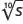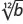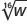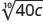Elementary Algebra

# 9.8Rational Exponents

Elementary Algebra9.8 Rational Exponents

### Learning Objectives

By the end of this section, you will be able to:

• Simplify expressions with $a1na1n$
• Simplify expressions with $amnamn$
• Use the Laws of Exponents to simply expressions with rational exponents

### Be Prepared 9.8

Before you get started, take this readiness quiz.

1. Add: $715+512715+512$.
If you missed this problem, review Example 1.81.
2. Simplify: $(4x2y5)3(4x2y5)3$.
If you missed this problem, review Example 6.24.
3. Simplify: $5−35−3$.
If you missed this problem, review Example 6.89.

### Simplify Expressions with $a1na1n$

Rational exponents are another way of writing expressions with radicals. When we use rational exponents, we can apply the properties of exponents to simplify expressions.

The Power Property for Exponents says that $(am)n=am·n(am)n=am·n$ when m and n are whole numbers. Let’s assume we are now not limited to whole numbers.

Suppose we want to find a number p such that $(8p)3=8(8p)3=8$. We will use the Power Property of Exponents to find the value of p.

$(8p)3=8Multiply the exponents on the left.83p=8Write the exponent 1 on the right.83p=81The exponents must be equal.3p=1Solve forp.p=13So(813)3=8.(8p)3=8Multiply the exponents on the left.83p=8Write the exponent 1 on the right.83p=81The exponents must be equal.3p=1Solve forp.p=13So(813)3=8.$

But we know also $(83)3=8(83)3=8$. Then it must be that $813=83813=83$.

This same logic can be used for any positive integer exponent n to show that $a1n=ana1n=an$.

### Rational Exponent $a 1 n a 1 n$

If $anan$ is a real number and $n≥2n≥2$, $a1n=ana1n=an$.

There will be times when working with expressions will be easier if you use rational exponents and times when it will be easier if you use radicals. In the first few examples, you’ll practice converting expressions between these two notations.

### Example 9.103

Write as a radical expression: $x12x12$ $y13y13$ $z14z14$.

### Try It 9.205

Write as a radical expression: $t12t12$ $m13m13$ $r14r14$.

### Try It 9.206

Write as a radial expression: $b12b12$ $z13z13$ $p14p14$.

### Example 9.104

Write with a rational exponent: $xx$ $y3y3$ $z4z4$.

### Try It 9.207

Write with a rational exponent: $ss$ $x3x3$ $b4b4$.

### Try It 9.208

Write with a rational exponent: $vv$ $p3p3$ $p4p4$.

### Example 9.105

Write with a rational exponent: $5y5y$ $4x34x3$ $35z435z4$.

### Try It 9.209

Write with a rational exponent: $10m10m$ $3n53n5$ $36y436y4$.

### Try It 9.210

Write with a rational exponent: $3k73k7$ $5j45j4$ $82a382a3$.

In the next example, you may find it easier to simplify the expressions if you rewrite them as radicals first.

### Example 9.106

Simplify: $25122512$ $64136413$ $2561425614$.

### Try It 9.211

Simplify: $36123612$ $813813$ $16141614$.

### Try It 9.212

Simplify: $1001210012$ $27132713$ $81148114$.

Be careful of the placement of the negative signs in the next example. We will need to use the property $a−n=1ana−n=1an$ in one case.

### Example 9.107

Simplify: $(−64)13(−64)13$ $−6413−6413$ $(64)−13(64)−13$.

### Try It 9.213

Simplify: $(−125)13(−125)13$ $−12513−12513$ $(125)−13(125)−13$.

### Try It 9.214

Simplify: $(−32)15(−32)15$ $−3215−3215$ $(32)−15(32)−15$.

### Example 9.108

Simplify: $(−16)14(−16)14$ $−1614−1614$ $(16)−14(16)−14$.

### Try It 9.215

Simplify: $(−64)12(−64)12$ $−6412−6412$ $(64)−12(64)−12$.

### Try It 9.216

Simplify: $(−256)14(−256)14$ $−25614−25614$ $(256)−14(256)−14$.

### Simplify Expressions with $amnamn$

Let’s work with the Power Property for Exponents some more.

Suppose we raise $a1na1n$ to the power m.

$(a1n)mMultiply the exponents.a1n·mSimplify.amnSoamn=(an)m.(a1n)mMultiply the exponents.a1n·mSimplify.amnSoamn=(an)m.$

Now suppose we take $amam$ to the $1n1n$ power.

$(am)1nMultiply the exponents.am·1nSimplify.amnSoamn=amnalso.(am)1nMultiply the exponents.am·1nSimplify.amnSoamn=amnalso.$

Which form do we use to simplify an expression? We usually take the root first—that way we keep the numbers in the radicand smaller.

### Rational Exponent $a m n a m n$

For any positive integers m and n,

$amn=(an)mamn=amnamn=(an)mamn=amn$

### Example 9.109

Write with a rational exponent: $y3y3$ $x23x23$ $z34z34$.

### Try It 9.217

Write with a rational exponent: $x5x5$ $z34z34$ $y25y25$.

### Try It 9.218

Write with a rational exponent: $a25a25$ $b73b73$ $m54m54$.

### Example 9.110

Simplify: $932932$ $1252312523$ $81348134$.

### Try It 9.219

Simplify: $432432$ $27232723$ $6253462534$.

### Try It 9.220

Simplify: $853853$ $81328132$ $16341634$.

Remember that $b−p=1bpb−p=1bp$. The negative sign in the exponent does not change the sign of the expression.

### Example 9.111

Simplify: $16−3216−32$ $32−2532−25$ $4−524−52$.

### Try It 9.221

Simplify: $8−538−53$ $81−3281−32$ $16−3416−34$.

### Try It 9.222

Simplify: $4−324−32$ $27−2327−23$ $625−34625−34$.

### Example 9.112

Simplify: $−2532−2532$ $−25−32−25−32$ $(−25)32(−25)32$.

### Try It 9.223

Simplify: $−1632−1632$ $−16−32−16−32$ $(−16)−32(−16)−32$.

### Try It 9.224

Simplify: $−8132−8132$ $−81−32−81−32$ $(−81)−32(−81)−32$.

### Use the Laws of Exponents to Simplify Expressions with Rational Exponents

The same laws of exponents that we already used apply to rational exponents, too. We will list the Exponent Properties here to have them for reference as we simplify expressions.

### Summary of Exponent Properties

If $a,ba,b$ are real numbers and $m,nm,n$ are rational numbers, then

$Product Propertyam·an=am+nPower Property(am)n=am·nProduct to a Power(ab)m=ambmQuotient Propertyaman=am−n,a≠0,m>naman=1an−m,a≠0,n>mZero Exponent Definitiona0=1,a≠0Quotient to a Power Property(ab)m=ambm,b≠0Product Propertyam·an=am+nPower Property(am)n=am·nProduct to a Power(ab)m=ambmQuotient Propertyaman=am−n,a≠0,m>naman=1an−m,a≠0,n>mZero Exponent Definitiona0=1,a≠0Quotient to a Power Property(ab)m=ambm,b≠0$

When we multiply the same base, we add the exponents.

### Example 9.113

Simplify: $212·252212·252$ $x23·x43x23·x43$ $z34·z54z34·z54$.

### Try It 9.225

Simplify: $323·343323·343$ $y13·y83y13·y83$ $m14·m34m14·m34$.

### Try It 9.226

Simplify: $535·575535·575$ $z18·z78z18·z78$ $n27·n57n27·n57$.

We will use the Power Property in the next example.

### Example 9.114

Simplify: $(x4)12(x4)12$ $(y6)13(y6)13$ $(z9)23(z9)23$.

### Try It 9.227

Simplify: $(p10)15(p10)15$ $(q8)34(q8)34$ $(x6)43(x6)43$.

### Try It 9.228

Simplify: $(r6)53(r6)53$ $(s12)34(s12)34$ $(m9)29(m9)29$.

The Quotient Property tells us that when we divide with the same base, we subtract the exponents.

### Example 9.115

Simplify: $x43x13x43x13$ $y34y14y34y14$ $z23z53z23z53$.

### Try It 9.229

Simplify: $u54u14u54u14$ $v35v25v35v25$ $x23x53x23x53$.

### Try It 9.230

Simplify: $c125c25c125c25$ $m54m94m54m94$ $d15d65d15d65$.

Sometimes we need to use more than one property. In the next two examples, we will use both the Product to a Power Property and then the Power Property.

### Example 9.116

Simplify: $(27u12)23(27u12)23$ $(8v14)23(8v14)23$.

### Try It 9.231

Simplify: $(32x13)35(32x13)35$ $(64y23)13(64y23)13$.

### Try It 9.232

Simplify: $(16m13)32(16m13)32$ $(81n25)32(81n25)32$.

### Example 9.117

Simplify: $(m3n9)13(m3n9)13$ $(p4q8)14(p4q8)14$.

We will use both the Product and Quotient Properties in the next example.

### Example 9.118

Simplify: $x34·x−14x−64x34·x−14x−64$ $y43·yy−23y43·yy−23$.

### Try It 9.233

Simplify: $m23·m−13m−53m23·m−13m−53$ $n16·nn−116n16·nn−116$.

### Try It 9.234

Simplify: $u45·u−25u−135u45·u−25u−135$ $v12·vv−72v12·vv−72$.

### Section 9.8 Exercises

#### Practice Makes Perfect

Simplify Expressions with $a1na1n$

In the following exercises, write as a radical expression.

524.

$x12x12$
$y13y13$
$z14z14$

525.

$r12r12$
$s13s13$
$t14t14$

526.

$u15u15$
$v19v19$
$w120w120$

527.

$g17g17$
$h15h15$
$j125j125$

In the following exercises, write with a rational exponent.

528.

$−x7−x7$
$y9y9$
$f5f5$

529.

$r8r8$$t4t4$

530.

$a3a3$$cc$

531.

$u5u5$
$vv$532.

$7c37c3$
$12d712d7$
$35f435f4$

533.

$5x45x4$
$9y89y8$
$73z573z5$

534.

$21p21p$
$8q48q4$
$436r6436r6$

535.

$25a325a3$
$3b3b$In the following exercises, simplify.

536.

$81128112$
$1251312513$
$64126412$

537.

$6251462514$
$2431524315$
$32153215$

538.

$16141614$
$16121612$
$312515312515$

539.

$2161321613$
$32153215$
$81148114$

540.

$(−216)13(−216)13$
$−21613−21613$
$(216)−13(216)−13$

541.

$(−243)15(−243)15$
$−24315−24315$
$(243)−15(243)−15$

542.

$(−1)13(−1)13$
$−113−113$
$(1)−13(1)−13$

543.

$(−1000)13(−1000)13$
$−100013−100013$
$(1000)−13(1000)−13$

544.

$(−81)14(−81)14$
$−8114−8114$
$(81)−14(81)−14$

545.

$(−49)12(−49)12$
$−4912−4912$
$(49)−12(49)−12$

546.

$(−36)12(−36)12$
$−3612−3612$
$(36)−12(36)−12$

547.

$(−1)14(−1)14$
$(1)−14(1)−14$
$−114−114$

548.

$(−100)12(−100)12$
$−10012−10012$
$(100)−12(100)−12$

549.

$(−32)15(−32)15$
$(243)−15(243)−15$
$−12513−12513$

Simplify Expressions with $amnamn$

In the following exercises, write with a rational exponent.

550.

$m5m5$
$n23n23$
$p34p34$

551.

$r74r74$
$s35s35$
$t73t73$

552.

$u25u25$
$v85v85$
$w49w49$

553.

$a3a3$
$b5b5$
$c53c53$

In the following exercises, simplify.

554.

$16321632$
$823823$
$10,0003410,00034$

555.

$100023100023$
$25322532$
$32353235$

556.

$27532753$
$16541654$
$32253225$

557.

$16321632$
$1255312553$
$64436443$

558.

$32253225$
$27−2327−23$
$25−3225−32$

559.

$64526452$
$81−3281−32$
$27−4327−43$

560.

$25322532$
$9−329−32$
$(−64)23(−64)23$

561.

$1003210032$
$49−5249−52$
$(−100)32(−100)32$

562.

$−932−932$
$−9−32−9−32$
$(−9)32(−9)32$

563.

$−6432−6432$
$−64−32−64−32$
$(−64)32(−64)32$

564.

$−10032−10032$
$−100−32−100−32$
$(−100)32(−100)32$

565.

$−4932−4932$
$−49−32−49−32$
$(−49)32(−49)32$

Use the Laws of Exponents to Simplify Expressions with Rational Exponents

In the following exercises, simplify.

566.

$458·4118458·4118$
$m712·m1712m712·m1712$
$p37·p187p37·p187$

567.

$652·612652·612$
$n210·n810n210·n810$
$q25·q135q25·q135$

568.

$512·572512·572$
$c34·c94c34·c94$
$d35·d25d35·d25$

569.

$1013·10531013·1053$
$x56·x76x56·x76$
$y118·y218y118·y218$

570.

$(m6)52(m6)52$
$(n9)43(n9)43$
$(p12)34(p12)34$

571.

$(a12)16(a12)16$
$(b15)35(b15)35$
$(c11)111(c11)111$

572.

$(x12)23(x12)23$
$(y20)25(y20)25$
$(z16)116(z16)116$

573.

$(h6)43(h6)43$
$(k12)34(k12)34$
$(j10)75(j10)75$

574.

$x72x52x72x52$
$y52y12y52y12$
$r45r95r45r95$

575.

$s115s65s115s65$
$z73z13z73z13$
$w27w97w27w97$

576.

$t125t75t125t75$
$x32x12x32x12$
$m138m58m138m58$

577.

$u139u49u139u49$
$r157r87r157r87$
$n35n85n35n85$

578.

$(9p23)52(9p23)52$
$(27q32)43(27q32)43$

579.

$(81r45)14(81r45)14$
$(64s37)16(64s37)16$

580.

$(16u13)34(16u13)34$
$(100v25)32(100v25)32$

581.

$(27m34)23(27m34)23$
$(625n83)34(625n83)34$

582.

$(x8y10)12(x8y10)12$
$(a9b12)13(a9b12)13$

583.

$(r8s4)14(r8s4)14$
$(u15v20)15(u15v20)15$

584.

$(a6b16)12(a6b16)12$
$(j9k6)23(j9k6)23$

585.

$(r16s10)12(r16s10)12$
$(u10v5)45(u10v5)45$

586.

$r52·r−12r−32r52·r−12r−32$
$s15·ss−95s15·ss−95$

587.

$a34·a−14a−104a34·a−14a−104$
$b23·bb−73b23·bb−73$

588.

$c53·c−13c−23c53·c−13c−23$
$d35·dd−25d35·dd−25$

589.

$m74·m−54m−24m74·m−54m−24$
$n37·nn−47n37·nn−47$

590.

$4 5 2 · 4 1 2 4 5 2 · 4 1 2$

591.

$n 2 6 · n 4 6 n 2 6 · n 4 6$

592.

$( a 24 ) 1 6 ( a 24 ) 1 6$

593.

$( b 10 ) 3 5 ( b 10 ) 3 5$

594.

$w 2 5 w 7 5 w 2 5 w 7 5$

595.

$z 2 3 z 8 3 z 2 3 z 8 3$

596.

$( 27 r 3 5 ) 1 3 ( 27 r 3 5 ) 1 3$

597.

$( 64 s 3 5 ) 1 6 ( 64 s 3 5 ) 1 6$

598.

$( r 9 s 12 ) 1 3 ( r 9 s 12 ) 1 3$

599.

$( u 12 v 18 ) 1 6 ( u 12 v 18 ) 1 6$

#### Everyday Math

600.

Landscaping Joe wants to have a square garden plot in his backyard. He has enough compost to cover an area of 144 square feet. Simplify $1441214412$ to find the length of each side of his garden.

601.

Landscaping Elliott wants to make a square patio in his yard. He has enough concrete to pave an area of 242 square feet. Simplify $2421224212$ to find the length of each side of his patio.Round to the nearest tenth of a foot.

602.

Gravity While putting up holiday decorations, Bob dropped a decoration from the top of a tree that is 12 feet tall. Simplify $1212161212121612$ to find how many seconds it took for the decoration to reach the ground. Round to the nearest tenth of a second.

603.

Gravity An airplane dropped a flare from a height of 1024 feet above a lake. Simplify $10241216121024121612$ to find how many seconds it took for the flare to reach the water.

#### Writing Exercises

604.

Show two different algebraic methods to simplify $432.432.$ Explain all your steps.

605.

Explain why the expression $(−16)32(−16)32$ cannot be evaluated.

Order a print copy

As an Amazon Associate we earn from qualifying purchases.